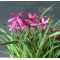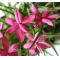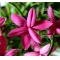##### The Japanese Bonsai specialist
Direct order Contact Help / Services Newsletter# Rhodohypoxis 'claudia' 1.4 litre pot

Rhodohypoxisref. : 9021

6,40

Available quantity : 31Order

###### Description

Vivacious plant, rhizomes. Leaves 40/50 mm. Delivered as a strong two-year old tuft in a plastic 12*12 cm pot.

At the start of summer the rhodohypoxis 'Claudia' spiky, slightly furry green leaves are surrounded by numerous 10/15 mm flowers in sumptuous colours. Flowering lasts for between 3 and 4 weeks. Dark pink colour. The foliage dies back in winter when it's best to keep the plant away from frosts in a cold greenhouse with almost no water.

#rhodohypoxis 4.2 #claudia 3.7 #kusamono 2.8 #leaves 2.6 #plants 2.6 #litre 2.5 #small 2.5 #plant 2.5 #surrounded 2 #greenhouse 2

Formule
(( ROUND((CHAR_LENGTH(b.article_nom)-CHAR_LENGTH(REPLACE(b.article_nom, 'rhodohypoxis', '')))/LENGTH('rhodohypoxis')) + ROUND((CHAR_LENGTH(b.article_description)-CHAR_LENGTH(REPLACE(b.article_description, 'rhodohypoxis', '')))/LENGTH('rhodohypoxis')) ) * 4.2) + (( ROUND((CHAR_LENGTH(b.article_nom)-CHAR_LENGTH(REPLACE(b.article_nom, 'claudia', '')))/LENGTH('claudia')) + ROUND((CHAR_LENGTH(b.article_description)-CHAR_LENGTH(REPLACE(b.article_description, 'claudia', '')))/LENGTH('claudia')) ) * 3.7) + (( ROUND((CHAR_LENGTH(b.article_nom)-CHAR_LENGTH(REPLACE(b.article_nom, 'leaves', '')))/LENGTH('leaves')) + ROUND((CHAR_LENGTH(b.article_description)-CHAR_LENGTH(REPLACE(b.article_description, 'leaves', '')))/LENGTH('leaves')) ) * 2.6) + (( ROUND((CHAR_LENGTH(b.article_nom)-CHAR_LENGTH(REPLACE(b.article_nom, 'plant', '')))/LENGTH('plant')) + ROUND((CHAR_LENGTH(b.article_description)-CHAR_LENGTH(REPLACE(b.article_description, 'plant', '')))/LENGTH('plant')) ) * 2.5) + (( ROUND((CHAR_LENGTH(b.article_nom)-CHAR_LENGTH(REPLACE(b.article_nom, 'litre', '')))/LENGTH('litre')) + ROUND((CHAR_LENGTH(b.article_description)-CHAR_LENGTH(REPLACE(b.article_description, 'litre', '')))/LENGTH('litre')) ) * 2.5) + (( ROUND((CHAR_LENGTH(b.article_nom)-CHAR_LENGTH(REPLACE(b.article_nom, 'greenhouse', '')))/LENGTH('greenhouse')) + ROUND((CHAR_LENGTH(b.article_description)-CHAR_LENGTH(REPLACE(b.article_description, 'greenhouse', '')))/LENGTH('greenhouse')) ) * 2) + (( ROUND((CHAR_LENGTH(b.article_nom)-CHAR_LENGTH(REPLACE(b.article_nom, 'surrounded', '')))/LENGTH('surrounded')) + ROUND((CHAR_LENGTH(b.article_description)-CHAR_LENGTH(REPLACE(b.article_description, 'surrounded', '')))/LENGTH('surrounded')) ) * 2) + (( ROUND((CHAR_LENGTH(b.article_nom)-CHAR_LENGTH(REPLACE(b.article_nom, 'vivacious', '')))/LENGTH('vivacious')) + ROUND((CHAR_LENGTH(b.article_description)-CHAR_LENGTH(REPLACE(b.article_description, 'vivacious', '')))/LENGTH('vivacious')) ) * 1.9) + (( ROUND((CHAR_LENGTH(b.article_nom)-CHAR_LENGTH(REPLACE(b.article_nom, 'sumptuous', '')))/LENGTH('sumptuous')) + ROUND((CHAR_LENGTH(b.article_description)-CHAR_LENGTH(REPLACE(b.article_description, 'sumptuous', '')))/LENGTH('sumptuous')) ) * 1.9) + (( ROUND((CHAR_LENGTH(b.article_nom)-CHAR_LENGTH(REPLACE(b.article_nom, 'delivered', '')))/LENGTH('delivered')) + ROUND((CHAR_LENGTH(b.article_description)-CHAR_LENGTH(REPLACE(b.article_description, 'delivered', '')))/LENGTH('delivered')) ) * 1.9)

## Secure payment## Delivery

Our logistic partners :04 74 55 23 48
Pépinière MAILLOT-BONSAÏ
Le Bois Frazy
01990 RELEVANT - FRANCE
on appointment